﻿ 自抗扰控制的车辆电动助力转向系统回正控制
«上一篇文章快速检索 高级检索

 哈尔滨工程大学学报2020, Vol. 41Issue (7): 1066-1072  DOI: 10.11990/jheu.2019050570

### 引用本文LI Zhipeng, NA Shaodan, CHANG Zifan. Research on the return-to-center control of a vehicle's electric power steering system based on active disturbance rejection control[J]. Journal of Harbin Engineering University, 2020, 41(7): 1066-1072. DOI: 10.11990/jheu.201905057.### 文章历史

1. 东北林业大学 交通学院, 黑龙江 哈尔滨 150040;
2. 哈尔滨力盛达机电科技有限公司, 黑龙江 哈尔滨 150000

Research on the return-to-center control of a vehicle's electric power steering system based on active disturbance rejection control
LI Zhipeng 1, NA Shaodan 1, CHANG Zifan 2
1. School of Traffic and Transportation, Northeast Forestry University, Harbin 150040, China;
2. Harbin Lishengda Electromechanical Technology Co., Ltd., Harbin 150000, China
Abstract: During a vehicle's return-to-center control process, the steering wheel's return-to-center speed is unstable due to system changes and external interference. This study proposes a current control, return-to-center method of active disturbance rejection control (ADRC). A dynamic equation of the electric power steering (EPS) system was established in order to analyze the torques, e.g. the return-to-center and the steering wheel torque. A return-to-center current control strategy based on the steering wheel speed and resist torque was developed. A linear extended state observer (LESO) of the motor speed model was designed to observe and compensate the low-frequency disturbance caused by the changes of the pipe string damping and motor parameters in real time. The tracking differentiator (TD) was used in order to track the target current and improve the motor current characteristics. The bench and vehicle test showed that this method can accurately distinguish the steering and return-to-center states and ensure that the return-to-center speed of steering wheel is smooth and has a small residual angle of return-to-center control, without affecting the steering state.
Keywords: electric power steering    return-to-center control    DC motor    speed estimation    active disturbance rejection control    extended state observer    tracking differentiator    simulation analysis

1 车辆转向系统动力学分析 1.1 车辆转向系统简化模型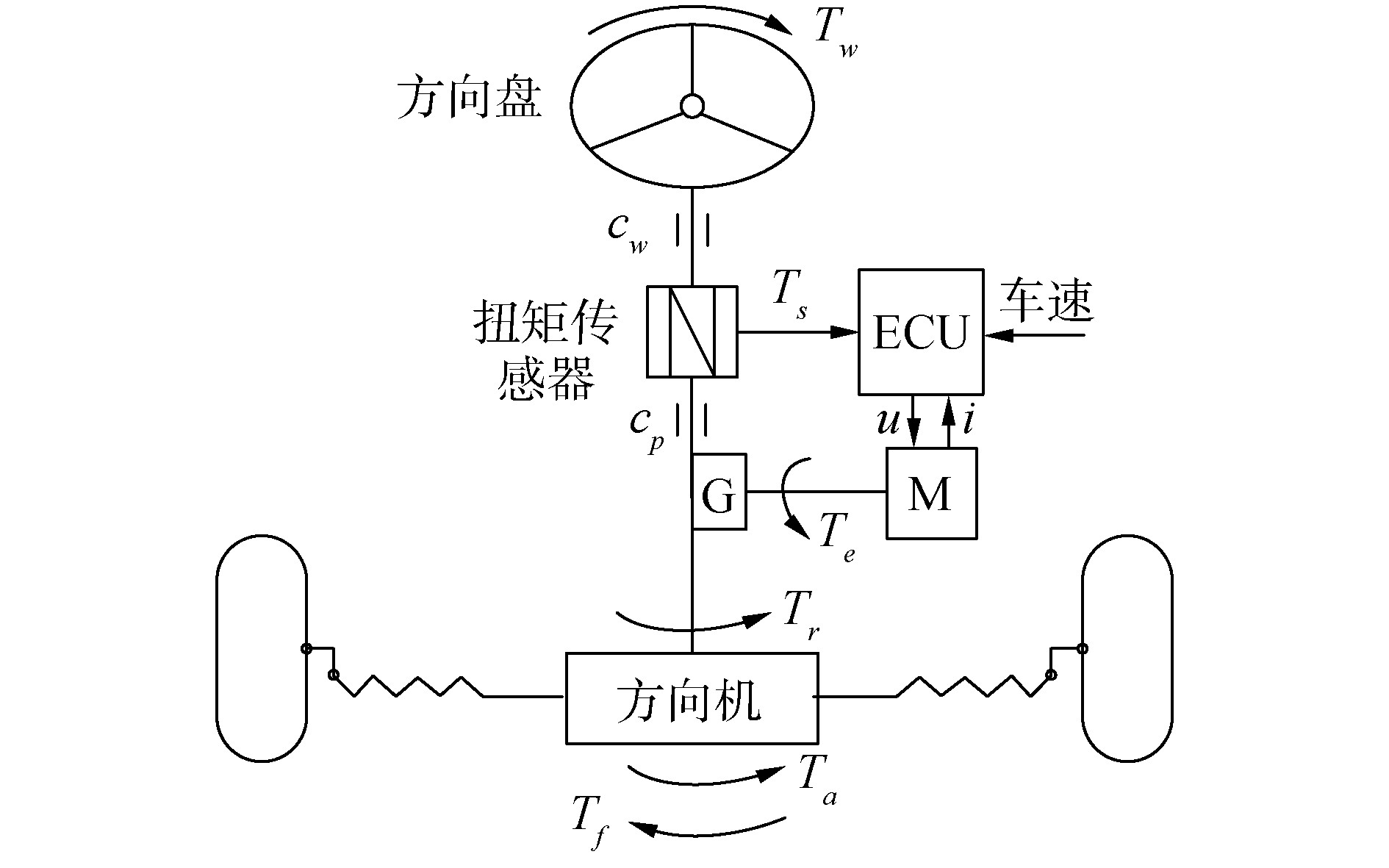Download: 图 1 车辆转向系统简化模型 Fig. 1 Simplified model of vehicle steering system

 ${T_w} - {T_s} = {J_w}{\dot \omega _w} + {c_w}{\omega _w}$ (1)

 ${T_w} \approx {T_s}$ (2)

 ${N_1}{T_e} + {T_s} = {T_r} + {J_p}{\dot \omega _p} + {c_p}{\omega _p}$ (3)

 ${T_r} = {T_a} + {T_f} {\rm{sgn}} ({\omega _p})$ (4)

 ${T_e} = {K_m}{i_a}$ (5)

 ${\omega _m} = {N_1}{\omega _p}$ (6)

 ${T_r} = {N_1}{K_m}{i_a} + {T_s} - {J_p}\frac{{{{\dot \omega }_m}}}{{{N_1}}} - {c_p}\frac{{{\omega _m}}}{{{N_1}}}$ (7)

 ${u_m} = {R_m}{i_a} + {K_e}{\omega _m}$ (8)

 $\left\{ {\begin{array}{*{20}{l}} {{\omega _m} = \frac{{{u_m} - {R_m}{i_a}}}{{{K_e}}}}\\ {{{\dot \omega }_m} = {{\left( {\frac{{{u_m} - {R_m}{i_a}}}{{{K_e}}}} \right)}^\prime }} \end{array}} \right.$ (9)

1.2 系统运动状系统运动状态判断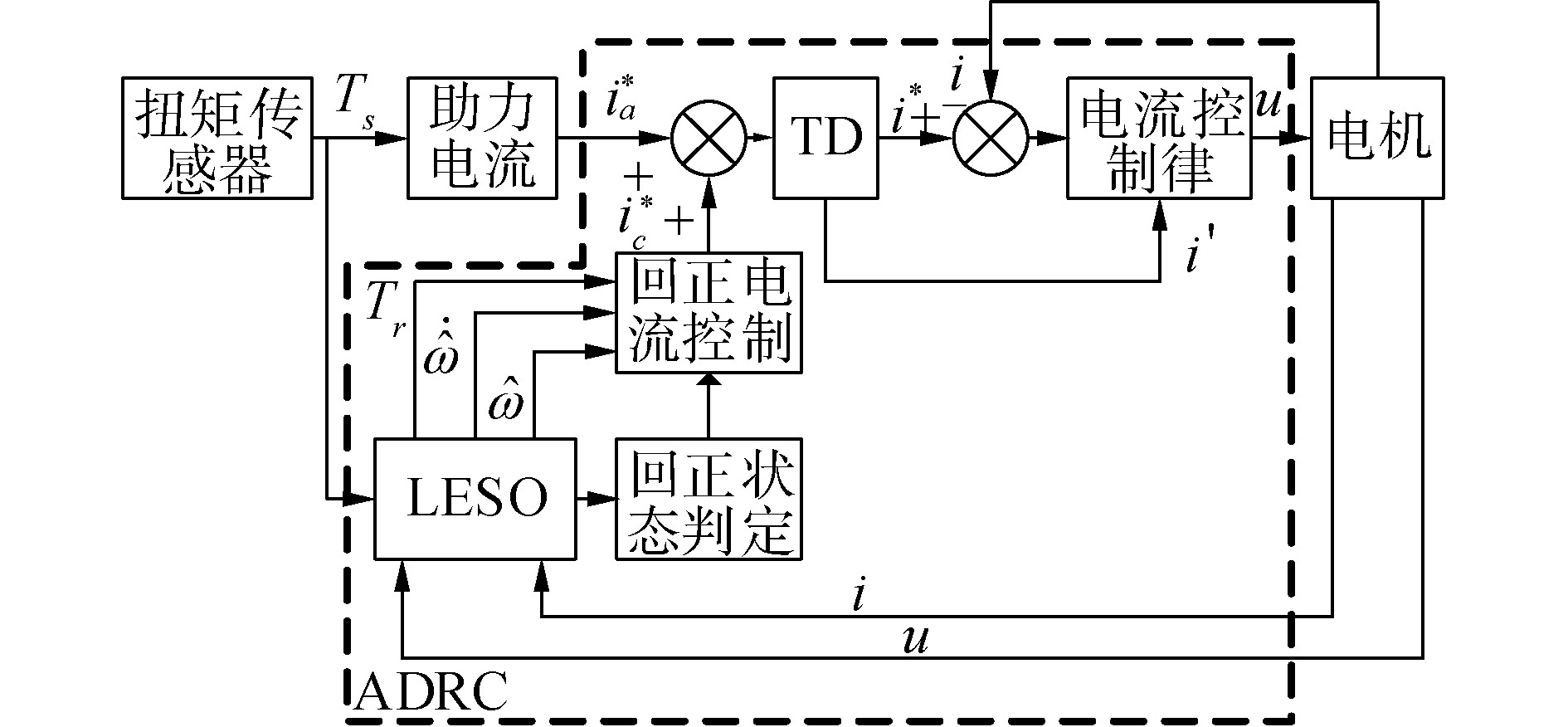Download: 图 2 电机控制逻辑框图 Fig. 2 Logic block diagram of motor control

2.1 主动回正控制律设计

 ${I_B} = \left\{ {\begin{array}{*{20}{l}} {{K_1}{T_r} - {K_2}({\omega _m} - {\omega _Y}),}&{{\omega _m} \ge {\omega _Y}}\\ {{K_1}{T_r},{\kern 1pt} {\kern 1pt} {\kern 1pt} {\kern 1pt} {\kern 1pt} {\kern 1pt} {\kern 1pt} {\kern 1pt} {\kern 1pt} {\kern 1pt} {\kern 1pt} {\kern 1pt} {\kern 1pt} {\kern 1pt} {\kern 1pt} {\kern 1pt} {\kern 1pt} {\omega _m} < {\omega _Y}}&{} \end{array}} \right.$ (10)

2.2 LESO的设计与稳定性分析

 $\left\{ {\begin{array}{*{20}{l}} {\dot \omega = \frac{1}{{{J_p}}}( - {c_p}\omega - {T_r} + {N_1}{K_m}{i_a})}\\ {\dot f = {f_k}}\\ {y = \omega } \end{array}} \right.$ (11)

 $f = - \frac{{{c_p}\omega + {T_r}}}{{{J_p}}}$ (12)

$\hat f$是总扰动估计值，eω为估计误差，β1β2是可调的反馈增益，b是输入增益，在实际应用中，非线性扩张观测器会极大的增加控制器的计算量，因此LESO应用得更为普遍。取误差反馈函数gn(eω)=eω表示系统模型，得到：

 $\left\{ \begin{array}{*{35}{l}} {{e}_{\omega }}=\omega -\hat{\omega } \\ \dot{\hat{\omega }}=\hat{f}+{{\beta }_{1}}{{e}_{\omega }}+{{i}_{a}} \\ \dot{\hat{f}}={{\beta }_{2}}{{e}_{\omega }} \\ \end{array} \right.$ (13)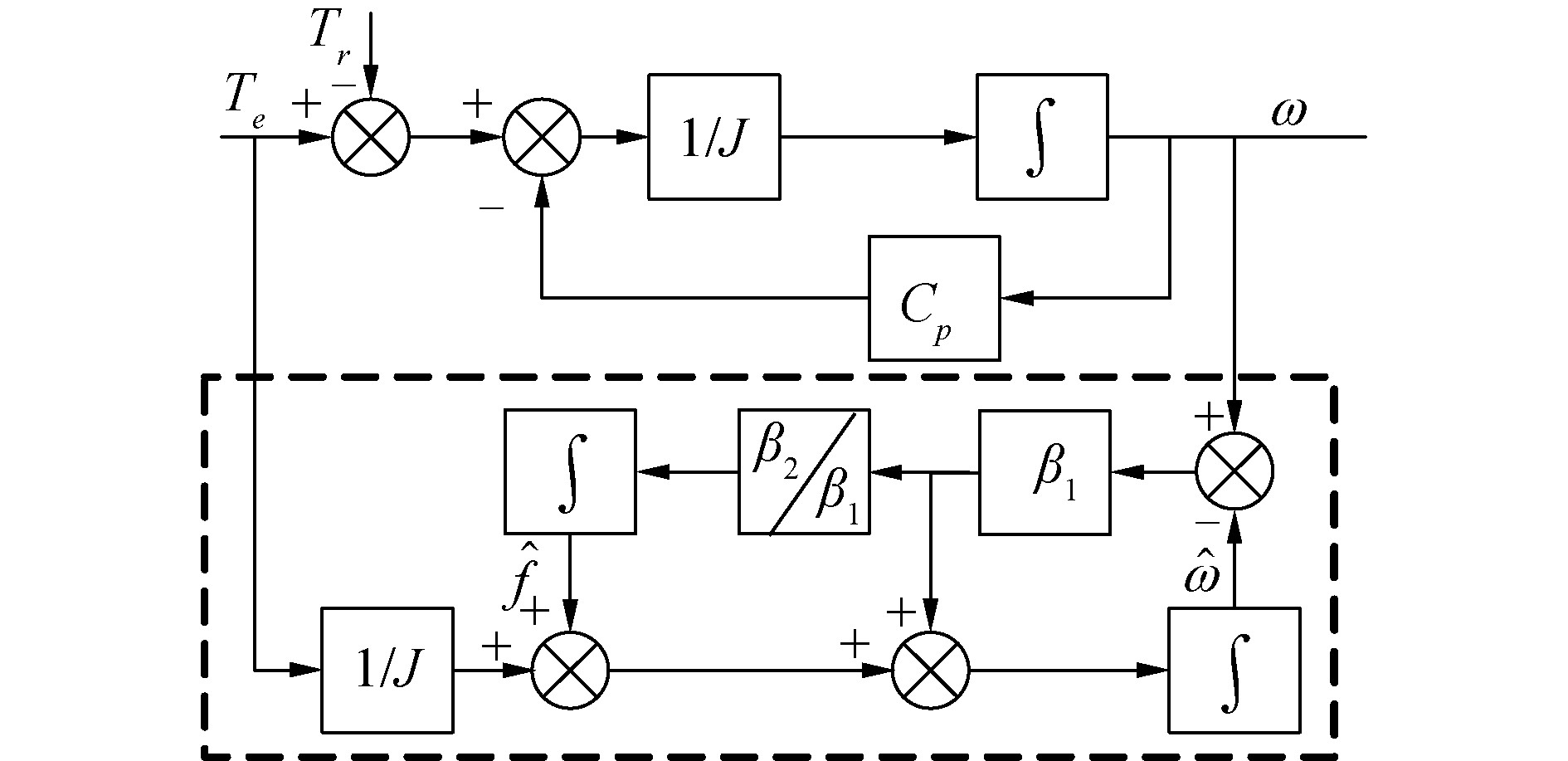Download: 图 3 LESO方框图 Fig. 3 LESO block diagram

 $\left[ \begin{matrix} {\dot{\hat{\omega }}} \\ {\dot{\hat{f}}} \\ \end{matrix} \right]=\left[ \begin{matrix} -{{\beta }_{1}} & 1 \\ -{{\beta }_{2}} & 0 \\ \end{matrix} \right]\left[ \begin{matrix} {\dot{\hat{\omega }}} \\ {\hat{f}} \\ \end{matrix} \right]+\left[ \begin{matrix} {{\beta }_{1}} \\ {{\beta }_{2}} \\ \end{matrix} \right]\omega +\left[ \begin{matrix} b \\ 0 \\ \end{matrix} \right]{{i}_{a}}$ (14)

 ${(s + {\delta _0})^2} = {s^2} + 2{\delta _0}s + \delta _0^2$ (15)

β2=δ02, β1=2δ0，代入LESO方程，假设初始状态为0时，拉普拉斯变换后，消去中间变量可得：

 $\frac{{\hat f(s)}}{{f(s)}} = \frac{{\delta _0^2}}{{{{(s + {\delta _0})}^2}}}$ (16)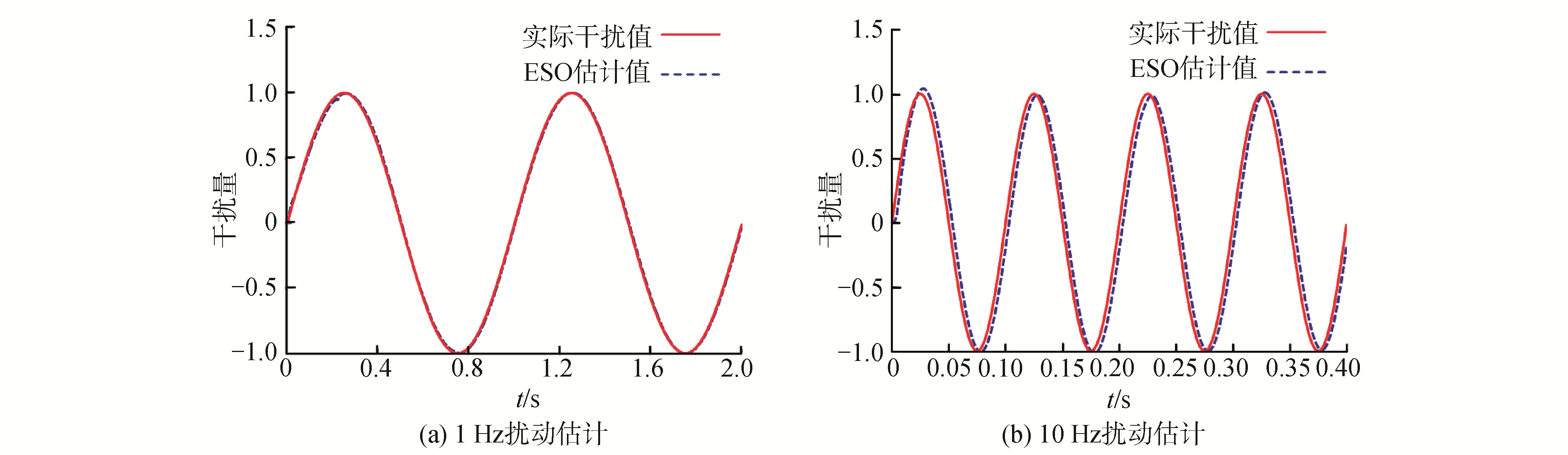Download: 图 4 LESO扰动估计结果 Fig. 4 The disturbance estimation results of the LESO

2.3 电流跟踪微分器设计

 $\left\{ {\begin{array}{*{20}{l}} {{{\mathit{\boldsymbol{\dot v}}}_1} = {\mathit{\boldsymbol{v}}_2}}\\ {{{\mathit{\boldsymbol{\dot v}}}_2} = u,|u| \le r} \end{array}} \right.$ (17)

 $\left\{ {\begin{array}{*{20}{l}} {{{\mathit{\boldsymbol{\dot v}}}_1} = {\mathit{\boldsymbol{v}}_2}}\\ {{{\mathit{\boldsymbol{\dot v}}}_2} = - r \cdot {\rm{sign}} \left( {{\mathit{\boldsymbol{v}}_1} - {\mathit{\boldsymbol{v}}_0} + \frac{{{\mathit{\boldsymbol{v}}_2}|{\mathit{\boldsymbol{v}}_2}|}}{{2r}}} \right),|u| \le r} \end{array}} \right.$ (18)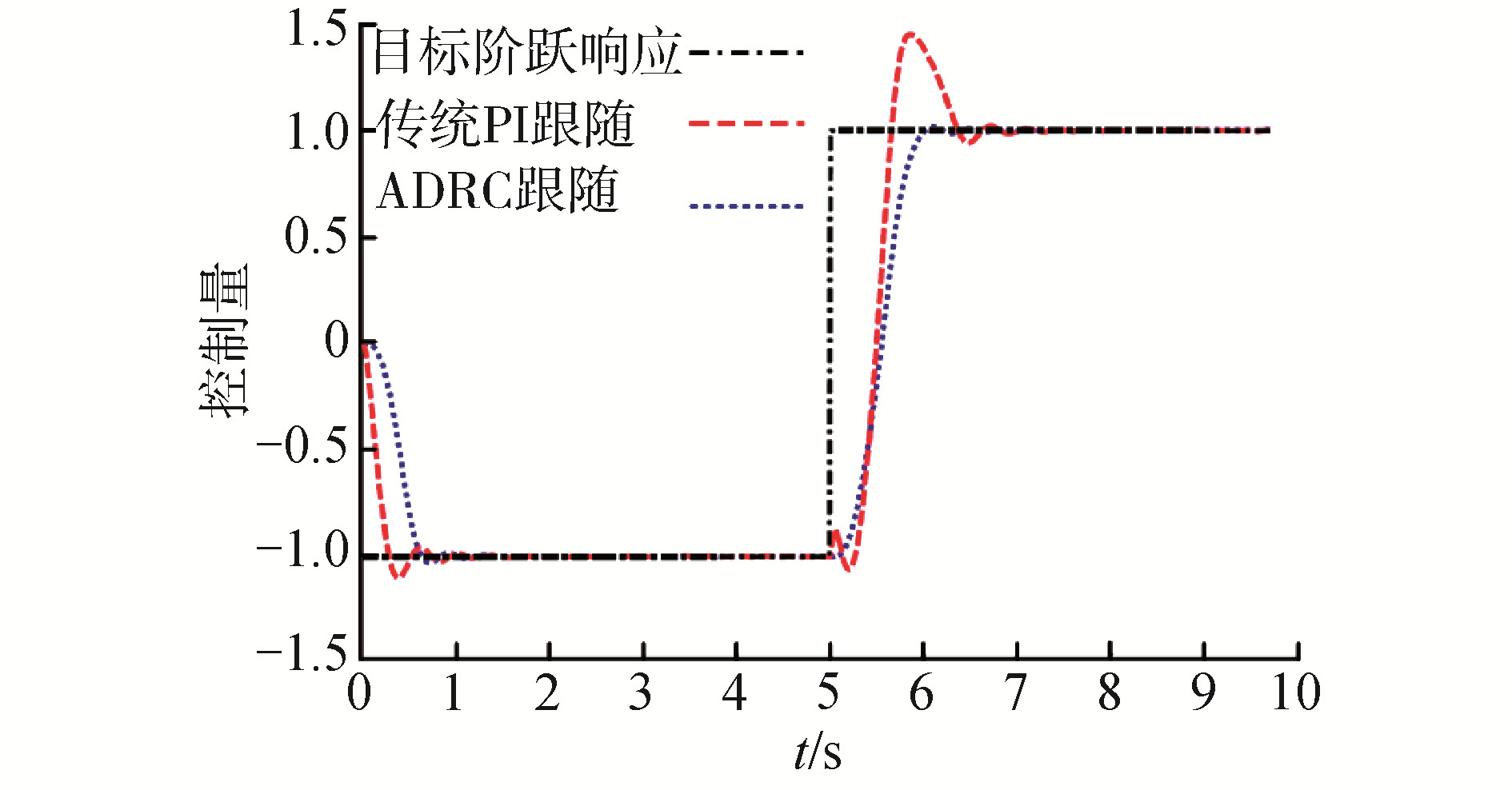Download: 图 5 系统阶跃响应跟随情况 Fig. 5 The results of the system step response

3 控制策略试验验证 3.1 台架实验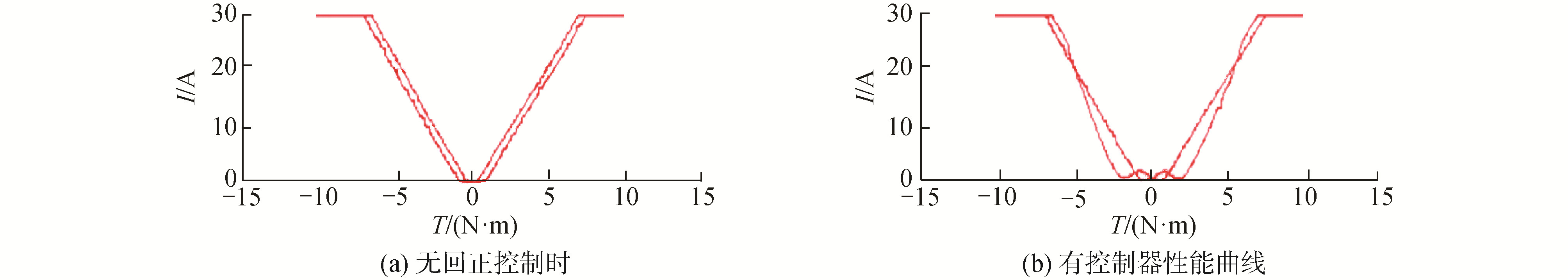Download: 图 6 试验控制器试验台性能曲线 Fig. 6 Test bed performance curve of the controller

3.2 实车实验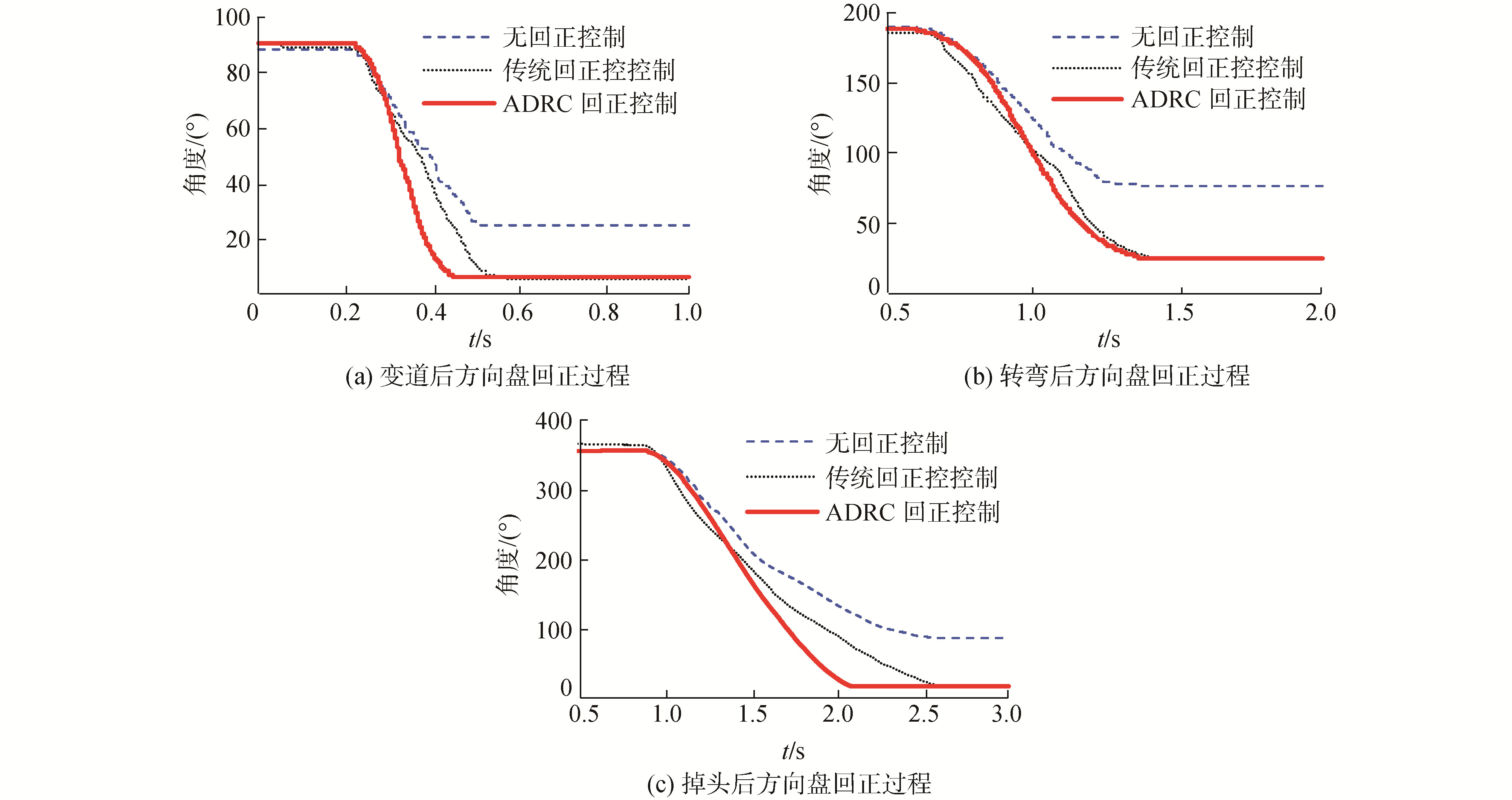Download: 图 7 方向盘角度变情况 Fig. 7 Variation of steering wheel angle表 1 实车试验结果 Table 1 The experimental results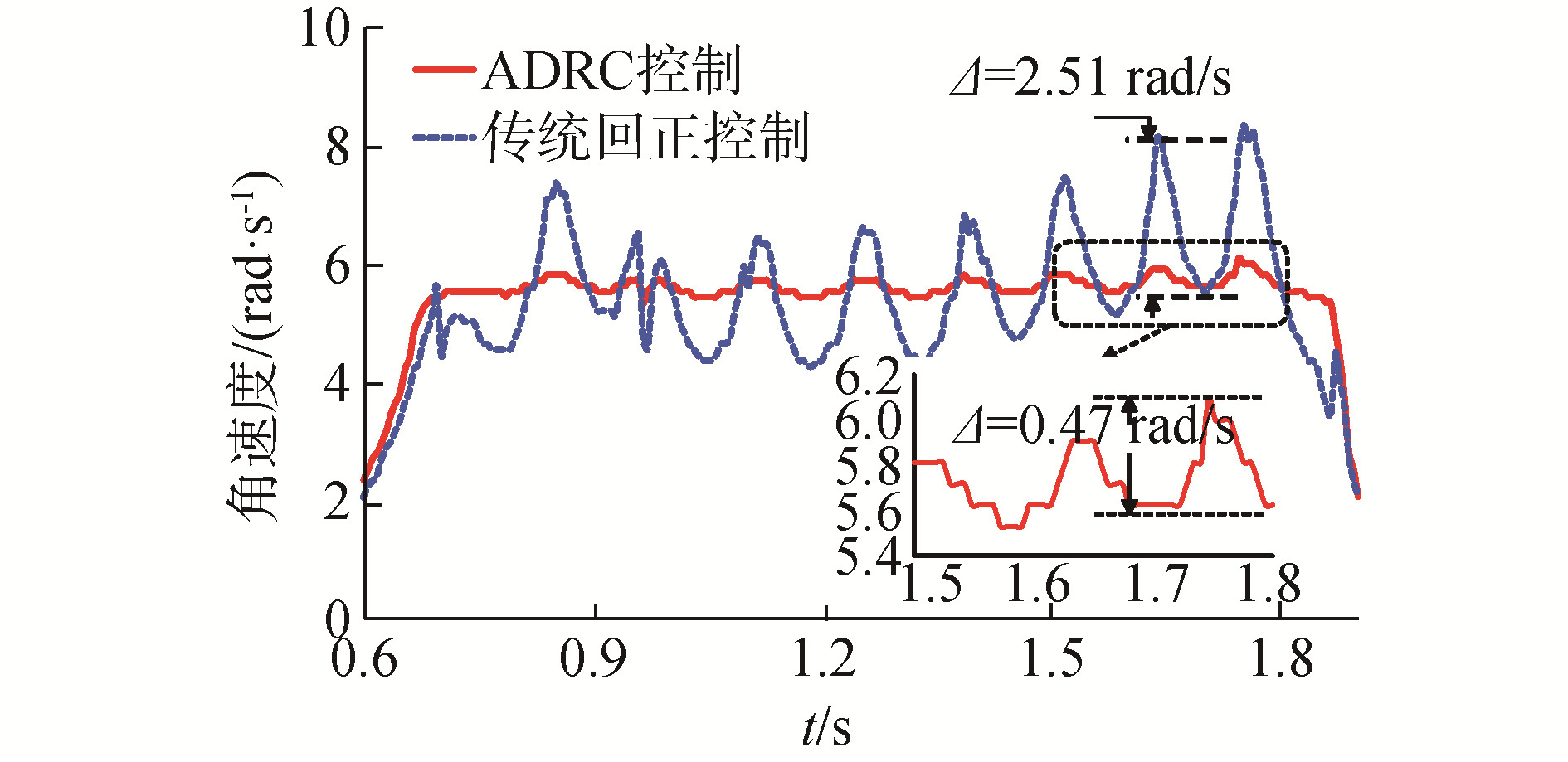Download: 图 8 方向盘角速度变化情况 Fig. 8 Variation of steering wheel angular velocity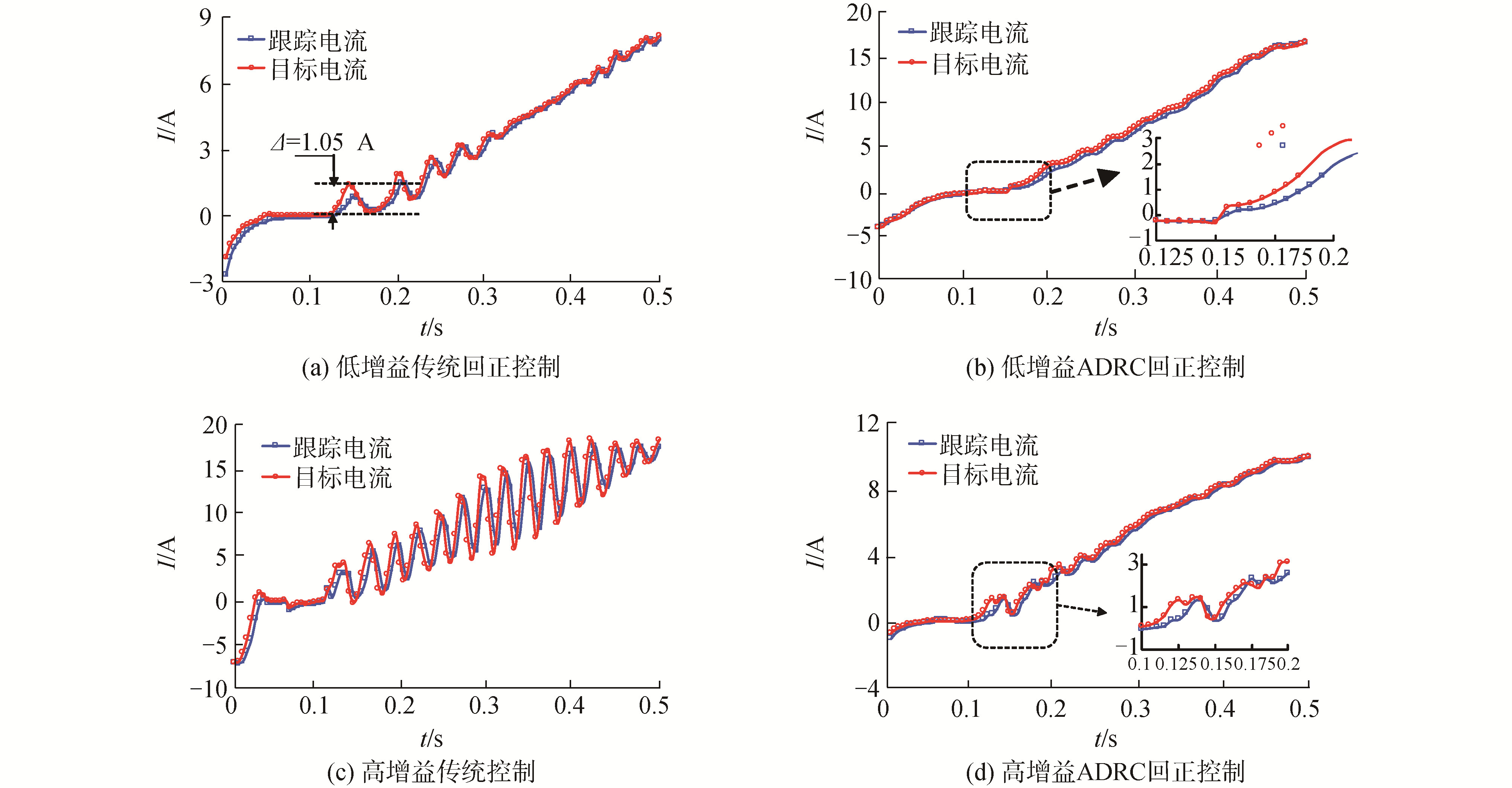Download: 图 9 回正过程电机电流 Fig. 9 Motor current during return state

4 结论

1) 提出方向盘回正时的EPS电机电流补偿方法，根据运动学方程估计管柱下端合力矩，以此确定系统回正状态。将方向盘角度和角速度信号反馈到回正控制策略可以提高车辆回正的稳定性。

  KIM J H, SONG J B. Control logic for an electric power steering system using assist motor[J]. Mechatronics, 2002, 12(3): 447-459. (0)  DANG Jianmin, CHEN Hui, GAO Bolin, et al. Optimal design of on-center steering force characteristic based on correlations between subjective and objective evaluations[J]. SAE international journal of passenger cars-mechanical systems, 2014, 7(3): 992-1001. DOI:10.4271/2014-01-0137 (0)  JO N H, JEON C, SHIM H. Noise reduction disturbance observer for disturbance attenuation and noise suppression[J]. IEEE transactions on industrial electronics, 2017, 64(2): 1381-1391. (0)  YANG Tao. A new control framework of electric power steering system based on admittance control[J]. IEEE transactions on control systems technology, 2015, 23(2): 762-769. (0)  陈久闪, 赵林峰, 祖春胜. 基于模糊非线性状态误差反馈策略的EPS回正控制[J]. 合肥工业大学学报(自然科学版), 2017, 40(5): 595-600. CHEN Jiushan, ZHAO Linfeng, ZU Chunsheng. EPS return control based on fuzzy NLSEF control method[J]. Journal of Hefei University of Technology (natural science), 2017, 40(5): 595-600. DOI:10.3969/j.issn.1003-5060.2017.05.004 (0)  MAROUF A, DJEMAI M, SENTOUH C, et al. A new control strategy of an electric-power-assisted steering system[J]. IEEE transactions on vehicular technology, 2012, 61(8): 3574-3589. (0)  FANKEM S, WEISKIRCHER T, MÜLLER S. Model-based rack force estimation for electric power steering[J]. IFAC proceedings volumes, 2014, 47(3): 8469-8474. (0)  SAIFIA D, CHADLI M, KARIMI H R, et al. Fuzzy control for Electric Power Steering system with assist motor current input constraints[J]. Journal of the franklin institute, 2015, 352(2): 562-576. DOI:10.1016/j.jfranklin.2014.05.007 (0)  CHABAAN R C, WANG Leyi. Control of electrical power assist systems:H∞ design, torque estimation and structural stability[J]. JSAE review, 2001, 22(4): 435-444. (0)  HAN Jingqing. From PID to active disturbance rejection control[J]. IEEE transactions on industrial electronics, 2009, 56(3): 900-906. (0)  CHEN Xiang, YANG Tiebao, CHEN Xiaoqun, et al. A generic model-based advanced control of electric power-assisted steering systems[J]. IEEE transactions on control systems technology, 2008, 16(6): 1289-1300. (0)  MA Xinlei, GUO Yongfeng, CHEN Lan. Active disturbance rejection control for electric power steering system with assist motor variable mode[J]. Journal of the franklin institute, 2018, 355(3): 1139-1155. (0)